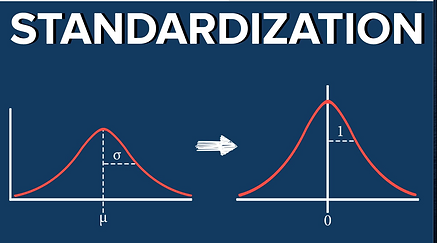top of page

## DATA CLEANING

Illustration of One Hot Encoding

1. Occasionally you may have to one hot encode or mask certain columns in a given data set in order to optimize accuracy and get the model to work.
1. One hot encoding essentially takes 2+ types of values in a column and makes them separate columns.
2. Masking simply converts a key word to a number.

## How to One Hot Encode?Standardization and Normalization

1. Conceptual

• When there are multiple inputs, there may be different scales or some inputs may be too large. In this scenario, standardization/normalization is used.

• Scaling makes the training of the model less sensitive to the scale of features so coefficients, such as the weights, can be solved for earlier

• This also helps to make sure that the cost does not converge and have a massive variance. In other words, simpler numbers helps get the weights with the least cost faster and efficiently.

• Helps compare different inputs with different units/scales

​​

1. When do I use Mean Normalization and when do I use Standardization?

• Normalization helps with varying scales and when the algorithm does not make assumptions about the distribution of data

• Standardization helps when your data has a bell curve distribution

2. When do I scale at all

• Whenever an algorithm computes cost or weights or/and when the scale of variables are very different, scale your inputs

3. Equations

• Standardizations equation involves utilizing Z-score to replace values

1.  X' = (X - mean)/Standard Deviation

2. The python way to do this would involve:

1. xStd = (x-  np.mean(x,axis = 0))/np.std(x,axis = 0)

3. The mean is now 0 and the standard deviation is now 1

• Normalization will result in a similar output and can be given by the equation

1. X' = (X - mean(x))/(max(x)-min(x))

2. They python way to do this would involve:

1. Xnorm = ((X - np.mean(x,axis=0))/(np.max(x,axis=0) - np.min(x,axis=0)))

3. Distribution of inputs are now -1 <= x’ <= 1

• An alternate scale called min-max scaling can also be used:

1. X' = (X - min(x))/(max(x)-min(x))

2. They python way to do this would involve:

1. Xnorm = ((X - np.min(x, axis = 0))/(np.max(x, axis = 0) - np.min(x, axis = 0)))

3. Distribution of inputs are now 0 <= x’ <= 1bottom of page